# Parabolic BounceDrop the ball on the curve, can you get it to hit the bell? Is there any position where it doesn’t hit the bell? Have you seen this shape anywhere before?
Maths

A parabola is a special shape in mathematics. It is the arc a projectile makes when thrown through the air.

What really makes a parabola special is that when a ball is drop onto the curve, the ball is reflected towards the focal point. This is true wherever you drop the ball.This property also works in reverse. Anything that originates from the focal point is reflected outwards, parallel to its line of symmetry.The equation of a parabola is y = ax2, where a is a constant. That means all parabolas are the same, except for scaling. Another way to define a parabola is to start with a horizonal line and a point above that line, and then to draw all the points that are an equal distance from the line and the point. A consequence of this definition will be that the focal point lies on every line of reflection.History

Parabolas have been studied since Ancient Greece, where it was found as a cross section of a cone. Diocles was the first Greek mathematician to prove the focal property of parabolas, which he used to focus sunlight to create a burning mirror.
Later, Italian astronomer Galileo Galilei proved the path of a projectile follows a parabola, a consequence of uniform acceleration due to gravity.
In 1663, Scottish astronomer and mathematician, James Gregory designed a telescope that used a parabolic mirror to focus the light. A parabolic mirror is a dish-shaped mirror, created by spinning a parabola around its line of symmetry.

PeopleDiocles c. 240BC – 180BC Diocles was a Greek mathematician. Little is known about Diocles except for his work. Diocles is thought to be the first to prove the focal property of parabolas and described using parabolic mirrors to focus sunlight to create a burning mirror. His work inspired later mathematicians who studied optics.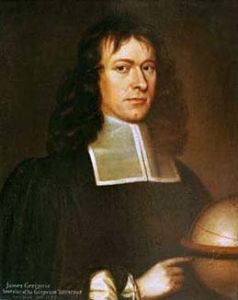James Gregory 1638 – 1675 James Gregory was a Scottish astronomer and mathematician. Originally from Aberdeen, Gregory became a professor of mathematics at St Andrews, and later at the University of Edinburgh. Gregory designed a type of reflecting telescope that used a parabolic mirror to focus the light. He is also known for his work in calculus, trigonometry and infinite series.
Applications

Parabolas appear in the physical world when throwing a projectile through the air, such as a ball or a jet of water.A parabolic dish is a dish shape made by spinning a parabola around its line of symmetry. Some types of telescopes use parabolic mirrors to focus the light onto one point. Similarly, satellite dishes and radio telescopes focus radio signals to one point.Car headlights use this idea in reverse by placing a light source at the focal point, which is then reflected outwards as a beam of light.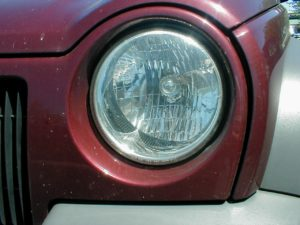Maths at Home

Here’s a way to make a parabola at home, using post-it notes (or similar).

1. Fold the paper in half to create a vertical line of symmetry. Place a dot somewhere on this line. This is the focal point.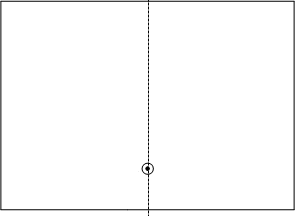2. Fold the bottom edge of the paper so that it touches the point. Make a crease and open the paper again.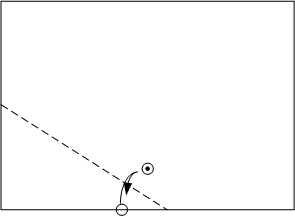3. Repeatedly fold, crease and open the paper at different spots along the edge.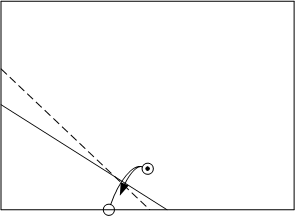4. If it is difficult to see your creases, try shading the reverse of each fold, or using wax paper. The many creases will form a parabola.This works because each point of the parabola you make is the same distance from the focal point as it is from the bottom edge. This is one of the definitions of a parabola.

How does changing the height of the focal point affect the parabola?